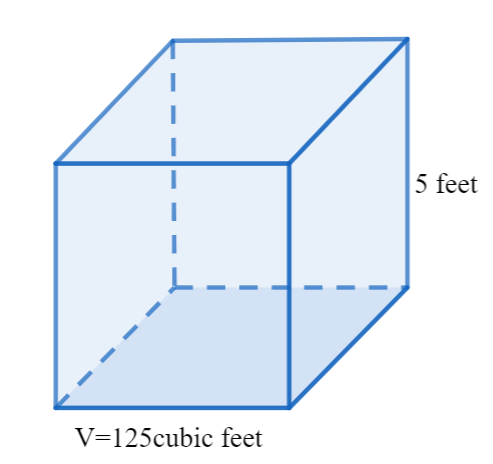Courses
Courses for Kids
Free study material
Offline Centres
MoreLast updated date: 06th Dec 2023
Total views: 280.5k
Views today: 3.80k

# A cube has a volume of 125 cubic feet, what is the length of each edge?Verified
280.5k+ views
Hint: In this problem, we have to find the length of each edge of a cube, whose given volume is 125 cubic feet. We know that the volume of the cube formula is $V={{a}^{3}}$, where a is the length. We already have the value of the volume, so we can equate the volume of the cube formula and the given value of the volume and simplify it. We can take the cube root on both sides and simplify it to get the length of each edge.

Here we have to find the length of each edge of a cube, whose given volume is 125 cubic feet.
We know that the volume of the cube formula is,
$V={{a}^{3}}$, where a is the length.
We already have the value of the volume which is 125 cubic feet, so we can equate the volume of the cube formula and the given value of the volume, we get
\begin{align} & \Rightarrow V=125 \\ & \Rightarrow {{a}^{3}}=125 \\ \end{align}
We can now take cubic root on both sides, we get
$\Rightarrow \sqrt{{{a}^{3}}}=\sqrt{125}$
We can now simplify the above term, we get
\begin{align} & \Rightarrow a=5 \\ & \because \sqrt{125}=5 \\ \end{align}Therefore, the length of each edge is 5 feet.

Note: We should always remember that the formula for volume of the cube is $V={{a}^{3}}$, where a is the length. We should know that, we can cancel the cubic root and the cube in the term, as the cubic root is one third and it can be canceled by 3 when multiplied to it.